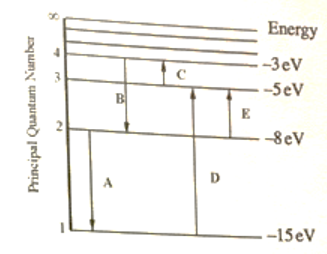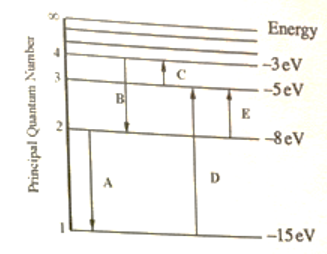# Problem: For this question consider the figure that shows an energy level diagram for a certain atom (not hydrogen). Several transitions are shown and are labeled by letters. Note: The diagram is not drawn to scale. Which transition corresponds to the emission of the photon with the longest wavelength? Which transition corresponds to the emission of the photon with the shortest wavelength?

###### FREE Expert Solution

We’re being asked to determine which transition results in the emission of a photon with the shortest and longest wavelength. Recall that starting from n = 1, the distance between each energy level gets smaller as shown below:Emission is a transition process from a higher energy level to a lower energy level. This rules out choices C, D and E. Recall that the energy of a photon is given by:

We can see that energy and frequency are directly proportional. The energy in a transition also depends on the distance between the energy levels.

98% (167 ratings)###### Problem Details

For this question consider the figure that shows an energy level diagram for a certain atom (not hydrogen). Several transitions are shown and are labeled by letters. Note: The diagram is not drawn to scale.Which transition corresponds to the emission of the photon with the longest wavelength?

Which transition corresponds to the emission of the photon with the shortest wavelength?